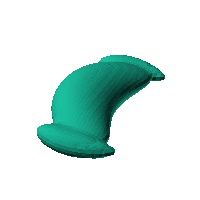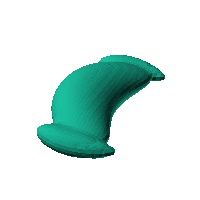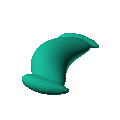Blob Farm# blob35The blob in all its glory:Designed as a potential hull for a spaceship.

 Click on the snapshot to download the blob's stl file.Octave Code:
```  # name of the blob
project = "blob35";

function w = f(x2,y2,z2,c,r,e)

cen1 = [50,140,0];  rx = 60; ry= 15; rz = 15; theta =1.1;
cen2 = [50,-140,0];
x  = (x2-c(1))/r(1);
y  = (y2-c(2))/r(2);
z  = (z2-c(3))/r(3);
# function at origin must be <0, and >0 far enough away.  w=0 defines the surface
c1 = [5,0,0];
r1 = [60,160,45];
w = 1./(((x-c1(1))/r1(1)).^2+((y-c1(2))/r1(2)).^2+((z-c1(3))/r1(3)).^2);

w = w+1./((y-cen1(2)).^2*(rx^2 + ry^2+(rx^2-ry^2)*cos(2*theta))/(2*rx^2*ry^2) ...
+ (x-cen1(1)).^2*(rx^2+ry^2+(ry^2-rx^2)*cos(2*theta))/(2*rx^2*ry^2) ...
+ (1/ry^2-(1/rx^2))*(x-cen1(1)).*(y-cen1(2))*sin(2*theta) ...
+ (((z-cen1(3))/rz).^2)).^2;

w = w+1./((y-cen2(2)).^2*(rx^2 + ry^2+(rx^2-ry^2)*cos(-2*theta))/(2*rx^2*ry^2) ...
+ (x-cen2(1)).^2*(rx^2+ry^2+(ry^2-rx^2)*cos(-2*theta))/(2*rx^2*ry^2) ...
+ (1/ry^2-(1/rx^2))*(x-cen2(1)).*(y-cen2(2))*sin(-2*theta) ...
+ (((z-cen2(3))/rz).^2)).^2;

w = 1-w;
endfunction;

# this is for distorting the grid before applying the function
# note that the undistorted grid will be used to make the stl file
# just set it to x3=x; y3=y; z3=z; if no warping is needed.
function [x3,y3,z3]= prewarp(x,y,z)
R =200;  # center of sphere is at (R+X0,0,0) with radius R (passing through (X0,0,0) )
X0 = 60; # this means that parts of the blob near [X0,0,0] will stay near that point.
# calculate the inverted coordinate of each point in the 3D grid (x3,y3,z3)
x2 = x-R-X0;  y2=y;   z2 = z; # intermediate values
r2 = R*R./(x2.^2+y2.^2+z2.^2);
x3 = x2.*r2+R+X0;  y3 = y2.*r2;  z3 = z2.*r2;
endfunction;

c_outer = [5,0,0];
r_outer = [1,1,1];

xmin = 40;
xmax = 180;
ymin = -200;
ymax = 200;
zmin = -50;
zmax = 50;

step = 4;  # grid pitch in mm  start with 4mm to see the shape quickly.  Once you have it just right, change to 2mm for printing

source("../octave/func2stl_v01.m");  # do all the calculations
```
GNU Octave